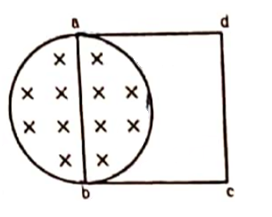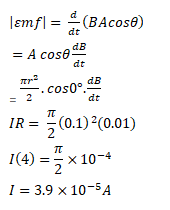# A uniform magnetic field B exists in a cylindrical region of radius 10 cm

Question:

A uniform magnetic field $B$ exists in a cylindrical region of radius $10 \mathrm{~cm}$ as shown in figure. A uniform wire of length $80 \mathrm{~cm}$ and resistance $4.0 \Omega$ is bent into a square frame and is placed with one side along a diameter of the cylindrical region. If the magnetic field increases at a constant rate of $0.010 \mathrm{~T} / \mathrm{s}$, find the current induced in the frame.Solution: